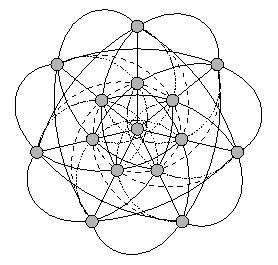´

## Construction of t-designs

A t-(v, k, lambda) designs is a pair D = (V, B) where

• V = {1, ..., v} is a set of points
• B = {B1, ..., Bb} is a set of k-subsets (blocks) of V with
• for every t-subset T of V there are exactly lambda blocks of the design containing T.
The parameter k is called the block size of the design, lambda is called the index. A famous example for a design is drawn in the following picture (click on the picture for further explanation):The parameters t, v, k and lambda have to fulfil certain restrictions to be admissible. It is admissible, if lambda is a multiple of a certain integer delta_lambda. You can find the detailed restrictions in almost every book on design theory. But they are not sufficient for the existence of designs with the given parameter set. So we have to find the designs quite explicitely. The designs are represented by solution vectors of systems of diophantine equations in the following manner:

For given t and k we consider the t- and k-subsets and we can build the incidence matrix M i.e. the rows of the matrix represent the t-subsets, the columns represent the k-subsets and the matrix entry mij is 1, if the i-th t-subset is incident with the j-th k-subset, otherwise 0. The search for t-(v, k, lambda) designs is equivalent to the search of 0/1-solutions of the system of equations

M · v = (lambda, ..., lambda)T
The block set of a design contains the j-th k-subset if and only if the j-th entry of the solution vector is 1. The problem with this method is the dimension of the matrix: we get (v choose t) rows and (v choose k) columns!

So we use the Kramer-Mesner method for the construction of t-designs with large t. That means the construction with prescribed automorphism group; we only consider orbit representatives of the orbits of the chosen group on the t-subsets and the k-subsets in the following way:

We can build the so called Kramer-Mesner matrix KM (instead of the full incidence matrix), where each row represents an orbit of t-subsets (with respect to the induced action of the group on the t-subsets) and each column analogously represents an orbit of k-subsets. So the entry kmij is a nonnegative integer c, where c is the number of elements of the j-th k-orbit containing a representative of the i-th t-orbit (one can show that c is well-defined).

This method is quite risky because we may choose the "wrong" permutation group, such that the number of solutions is 0 afterwards, although there are designs with the given parameter set but another (or just smaller) automorphism group! So we have to make a good guess for the group - for example the linear groups fairly often occur as automorphism groups of designs.

Now we have to solve much smaller systems of diophantine equations: the block set of a design with the prescribed group as an automorphism group is a union of whole orbits of k-subsets.

But we have to be careful:
the prescribed group usually is not the full automorphism group, but only a subgroup. So we can ask for the full automorphism group of the design(s) we have found. And it happens that, when you have found a bigger (probably the full) automorphism group, the orbits under the smaller group fuse under the action of the bigger group, such that you get less solutions (the question then is to identify the fusing designs).

Back to the DISCRETA-homepage.

Last updated: August 10, 1999, Evi Haberberger

University of Bayreuth -